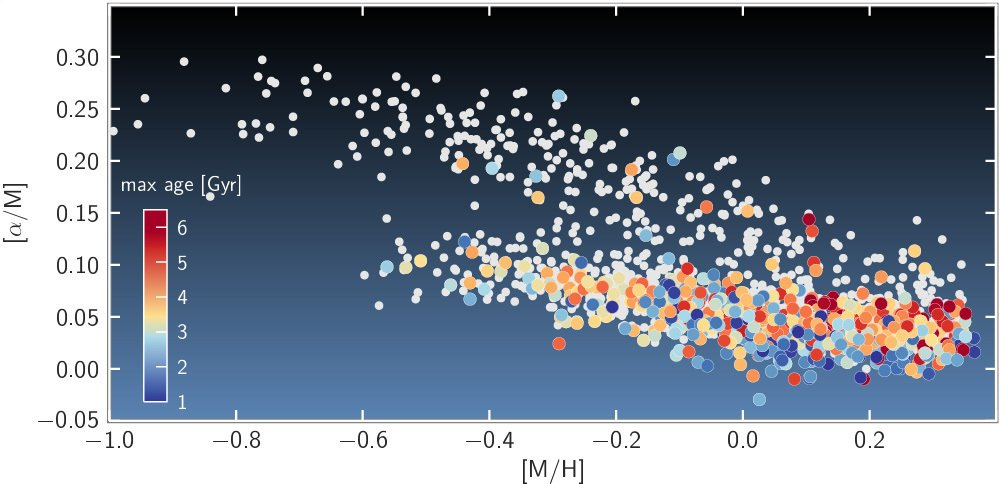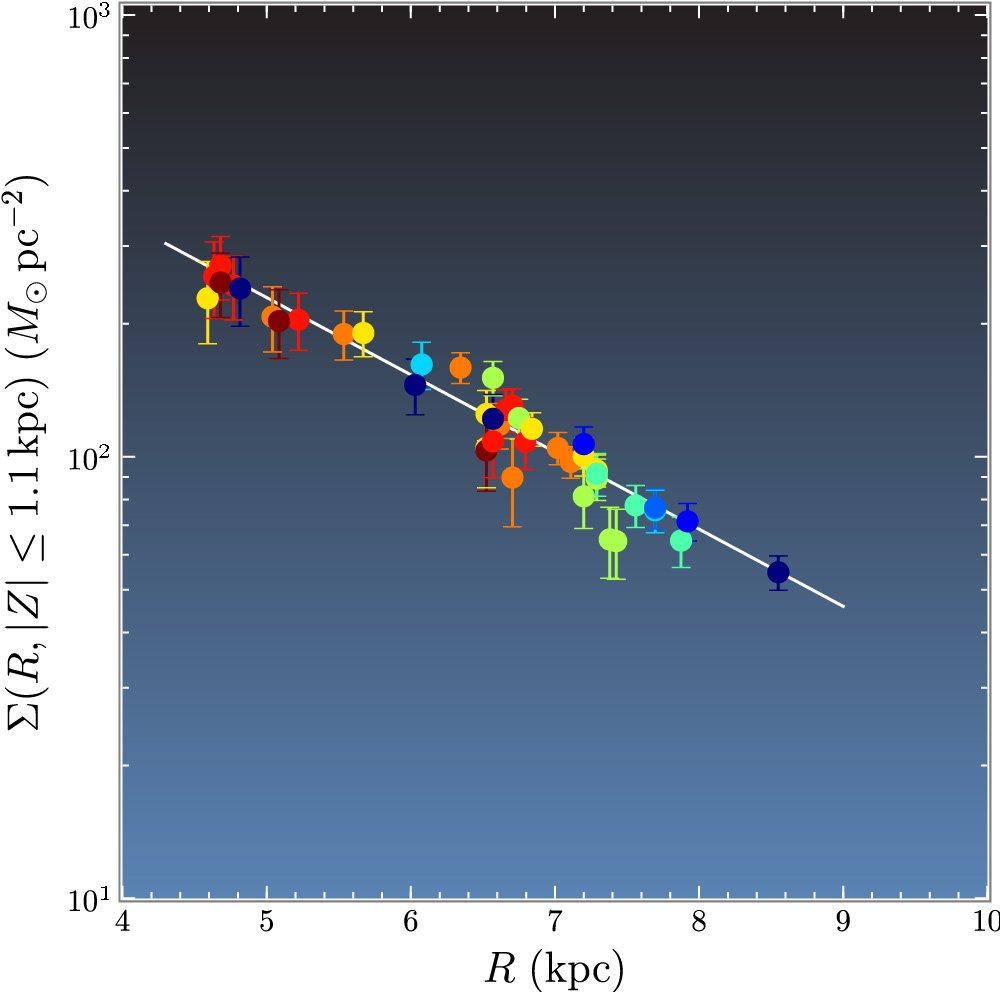# Dynamical ModelsZoom Image
Figure 7. Age and abundances for stars with seismic and spectroscopic information from Kepler and APOGEE. The plot shows the distribution of the sample in the [α/M] versus [M/H] plane. Small white dots represent stars for which ages are not measured (i.e., stars with a mass smaller than 1.2 M), and colored dots represent stars younger than 7 Gyr (the color encodes the maximal age of each star).Zoom Image
Surface density as a function of radius as measured by SEGUE G-dwarf mono-abundance stellar populations (MAPs). Each point represents a measurement based on the data from one MAP; each MAP measures the surface density Σ(R, |Z| 1.1 kpc) at the radius R where the correlation between the model stellar disk scale length and Σ(R0, |Z| 1.1 kpc) is minimized in the MAP’s PDF. The curve is an exponential fit with Σ(R0, |Z| 1.1 kpc) = 69 M pc−2 and a scale length of 2.5 kpc. Points are color-coded by the [α/Fe] of the MAP bin, from [α/Fe] = 0.025 (dark blue) to [α/Fe] = 0.475 (red/brown).

Dynamical models characterize the Milky Way gravitational potential based on stellar positions and motions.Go to Editor View